## RD Sharma Class 10 Solutions Chapter 10 Trigonometric Ratios Ex 10.3

These Solutions are part of RD Sharma Class 10 Solutions. Here we have given RD Sharma Class 10 Solutions Chapter 10 Trigonometric Ratios Ex 10.3

Other Exercises

Question 1.
Evaluate the following :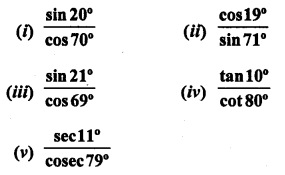Solution: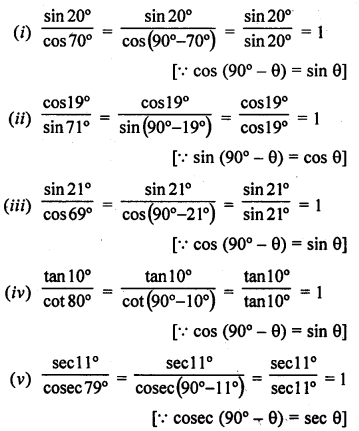Question 2.
Evaluate the following :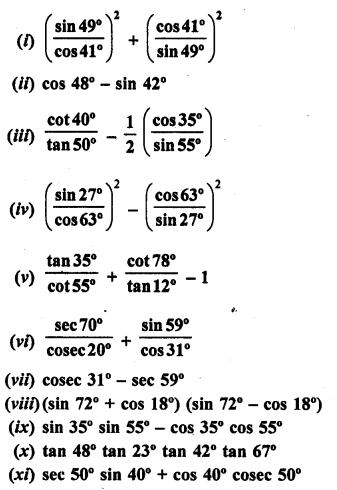Solution: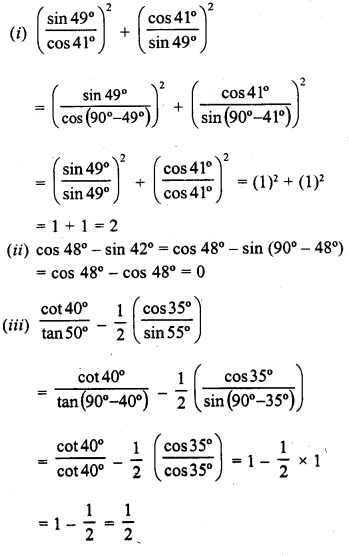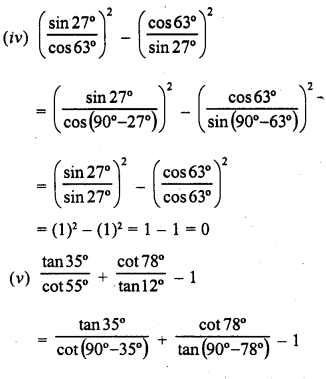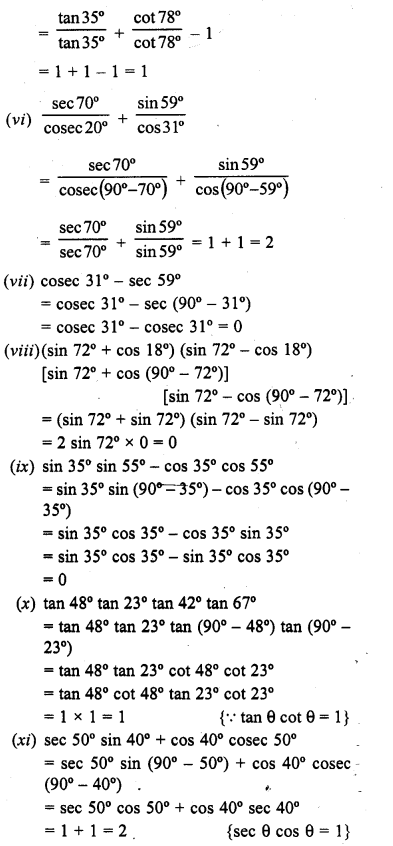Question 3.
Express each one of the following in terms of trigonometric ratios of angles lying between 0° and 45°
(i) sin 59° + cos 56°
(ii) tan 65° + cot 49“
(iii) sec 76° + cosec 52°
(iv) cos 78° + sec 78°
(v) cosec 54° + sin 72°
(vi) cot 85″ + cos 75°
(vii) sin 67° + cos 75°
Solution:
(i) sin 59° + cos 56°
= sin (90° – 31°) + cos (90° – 34°)
= cos 31° +sin 34°
(ii) tan 65° + cot 49°
= tan (90° – 25°) + cot (90° – 41°)
= cot 25° + tan 41°
(iii) sec 76° + cosec 52°
= sec (90° – 14°) + cosec (90 0 – 38°)
= cosec 14° + sec 38°
(iv) cos 78° + sec 78°
= cos (90° – 12°) + sec (90°- 12°)
= sin 12° + cosec 12°
(v) cosec 54° + sin 72°
= cosec (90° – 36°) + sin (90°-18°)
= sec 36° + cos 18°
(vi) cot 85° + cos 75°
= cot (90° – 5°) + cos (90° – 15°)
= tan 5° + sin 15°
(vii) sin 67° + cos 75°
= sin (90° – 23°) + cos (90° – 15°)
= cos 23° + sin 15°

Question 4.
Express cos 75° + cot 75° in terms of angles between 0° and 30°.
Solution:
cos 75° + cot 75° = cos (90° – 15°) + cot (90°-15°)
= sin 15° + tan 15°

Question 5.
If sin 3A = cos (A – 26°), where 3A is an acute angle, And the value of A.
Solution:
sin 3A = cos (A – 26°)
⇒ cos (90° – 3A) = cos (A – 26°)
Comparing,
90° – 3A = A – 26°
⇒ 90° + 26° = A + 3A ⇒ 4A = 116°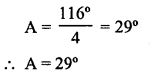Question 6.
If A, B, C are the interior angles of a triangle ABC, proveSolution: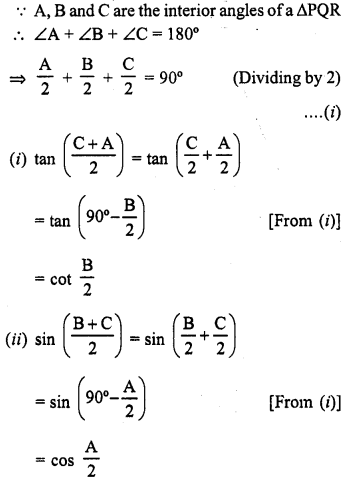Question 7.
Prove that :Solution: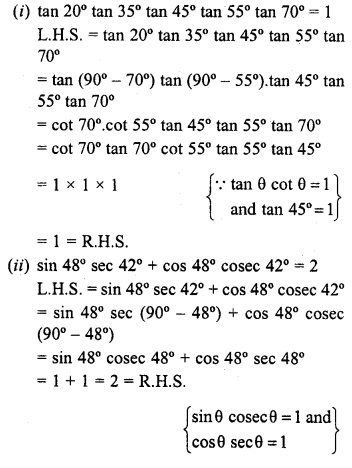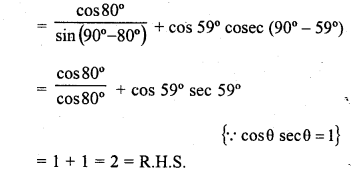Question 8.
Prove the following :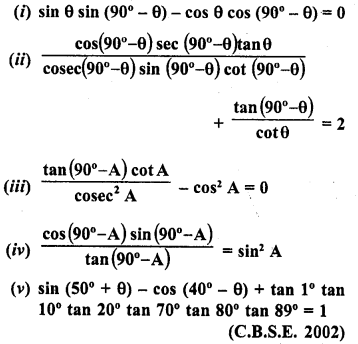Solution: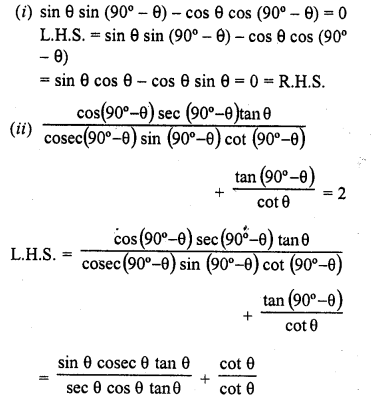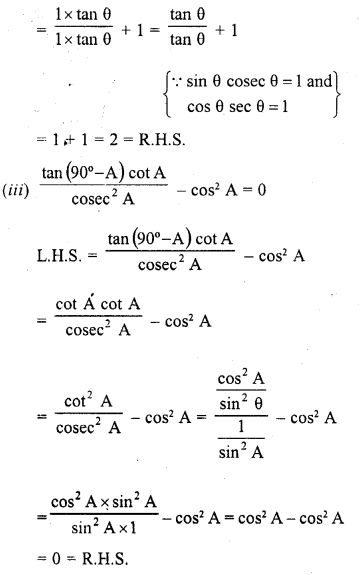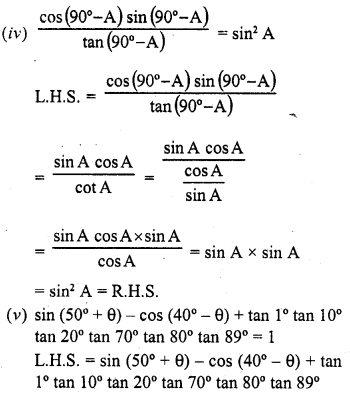Question 9.
Evaluate :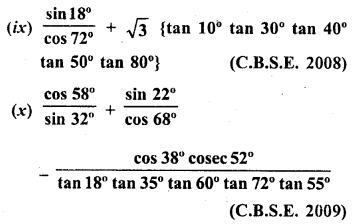Solution: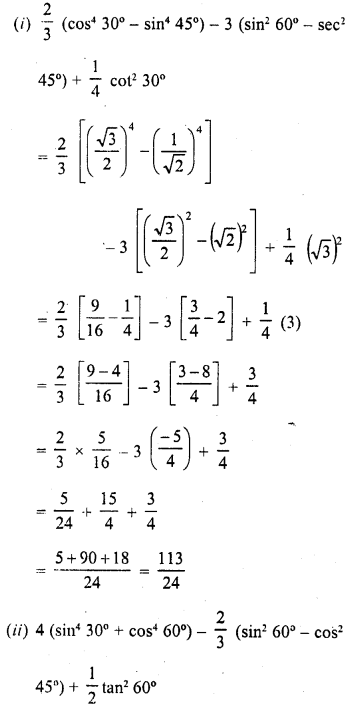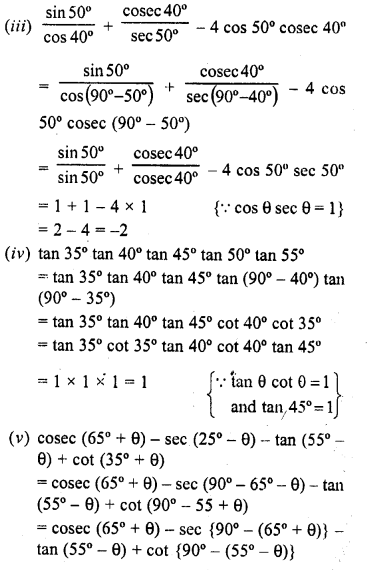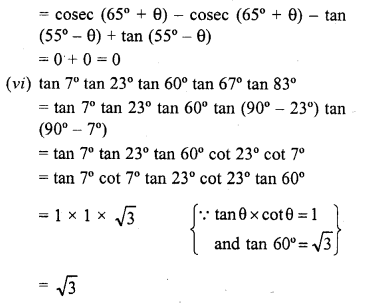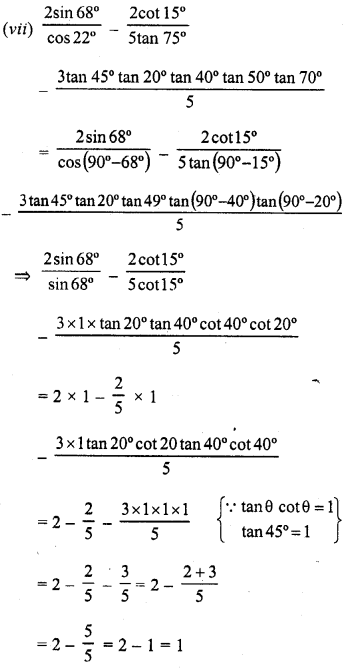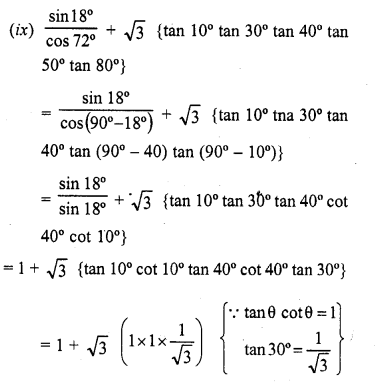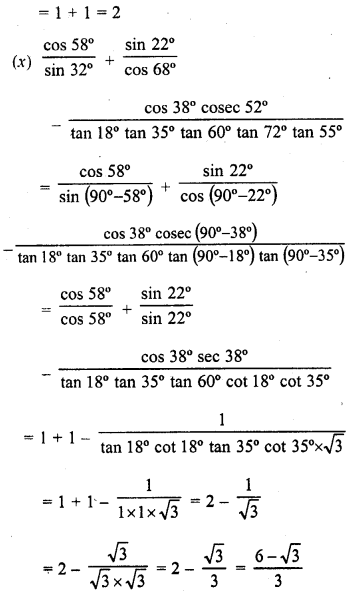Question 10.
If sin θ= cos (θ – 45°), where θ and (θ – 45°) are acute angles, find the degree measure of θ.
Solution:Question 11.
If A, B, C are the interior angles of a AABC, show that :
(i) $$sin\frac { B+C }{ 2 } cos\frac { A }{ 2 }$$
(ii) $$cos\frac { B+C }{ 2 } sin\frac { A }{ 2 }$$
Solution:Question 12.
If 2θ + 45° and 30° – θ are acute angles, find the degree measures of θ satisfying sin (20 + 45°) = cos (30° – θ).
Solution:Question 13.
If θ is a positive acute angle such that sec θ = cosec 60°, And the value of 2 cos2 θ-1.
Solution: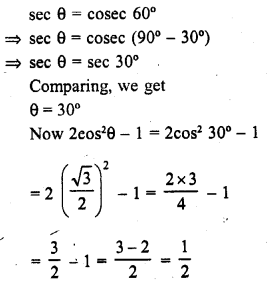Question 14.
If cos 2 θ – sin 4 θ, where 2 θ and 4 θ are acute angles, find the value of θ.
Solution: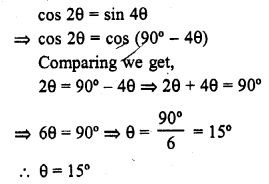Question 15.
If sin 3 θ = cos (θ – 6°), where 3 θ and θ – 6° are acute angles, find the value of θ.
Solution: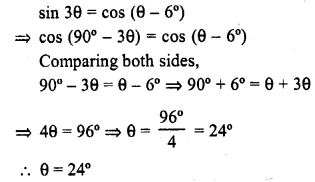Question 16.
If sec 4A = cosec (A – 20°), where 4A is an acute angle, find the value of A.
Solution: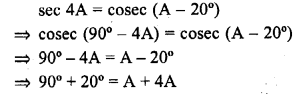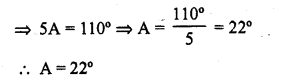Question 17.
If sec 2A = cosec (A – 42°), where 2A is an acute angle, find the value of A. (C.B.S.E. 2008)
Solution:Hope given RD Sharma Class 10 Solutions Chapter 10 Trigonometric Ratios Ex 10.3 are helpful to complete your math homework.

If you have any doubts, please comment below. Learn Insta try to provide online math tutoring for you.